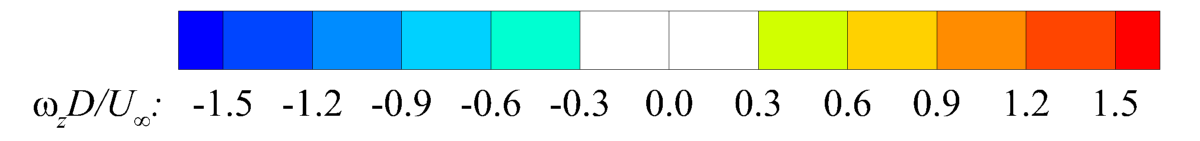## Movies

B. Zhang and C. Liang, "High-Order Numerical Simulation of Flows over Rotating Cylinders of Various Cross-Sectional Shapes", AIAA Paper, 2019-3430, June, 2019. doi:10.2514/6.2019-3430

1. Flow field around a rotating elliptic cylinder at $\dot{\alpha}=\pi/4$ and $Re=200$.
2. Flow field around a rotating triangular cylinder at $\dot{\alpha}=\pi/4$ and $Re=200$.
3. Flow field around a rotating square cylinder at $\dot{\alpha}=\pi/4$ and $Re=200$.
4. Flow field around a rotating circular cylinder at $\dot{\alpha}=\pi/4$ and $Re=200$.
5. Flow field when the elliptic cylinder rotates to its initial position.6. Flow field around a rotating elliptic cylinder at $\dot{\alpha}=\pi/4$ and $Re=1000$.
7. Flow field around a rotating triangular cylinder at $\dot{\alpha}=\pi/4$ and $Re=1000$.
8. Flow field around a rotating square cylinder at $\dot{\alpha}=\pi/4$ and $Re=1000$.
9. Flow field around a rotating circular cylinder at $\dot{\alpha}=\pi/4$ and $Re=1000$.
TOP